# Use Case 2: Particles¶

In this example we will subsample a dataset stored on SciServer using trajectories of synthetic Lagrangian particles. OceanSpy enables the extraction of properties at any given location, but it does not have functionality to compute Lagrangian trajectories yet. The trajectories used in this notebook have been previously computed using a Matlab-based Lagrangian particle code (Gelderloos et al., 2016). The Lagrangian particle code, along with synthetic oceanographic observational platforms (such as Argo floats, isobaric floats, current profiler, and gliders), will be incorporated into OceanSpy in future releases.

The animation below shows the change in temperature of synthetic particles along their 3D trajectories. Particles have been released in Denmark Strait and tracked backward in time from day 0 to day 180, and tracked forward in time from day 180 to 360. All particles are animated forward in time. Source: https://player.vimeo.com/video/296949375

:

# Display animation
from IPython.display import HTML

s = 'https://player.vimeo.com/video/296949375'

# Options
w = 640
h = 360
f = 0
a = 'autoplay; fullscreen'

# Display
HTML(f'<iframe src="{s}"'
'width="640" height="360" frameborder="0" allow="autoplay; fullscreen"'
'allowfullscreen></iframe>')

/home/idies/miniconda3/envs/Oceanography/lib/python3.7/site-packages/IPython/core/display.py:689: UserWarning: Consider using IPython.display.IFrame instead
warnings.warn("Consider using IPython.display.IFrame instead")

:

The subsampling method used in this notebook resembles Lagrangian floats deployed by observational oceanographers, such as RAFOS floats. RAFOS floats are isobaric and tracked acoustically. They measure water properties and provide accurate trajectories in both the horizontal and vertical dimensions. The following picture shows the deployment of a RAFOS float during the Overturning in the Subpolar North Atlantic Program (OSNAP). Source: www.o-snap.org## Extract¶

The computational time to extract particle properties depends on the number of particles, the length of their trajectories, the number of variables requested, and, more importantly, how far the particles travel in the domain and therefore, how dispersed the Lagrangian trajectories are. Thus, it is preferable to extract particle properties asynchronously using the Job mode of SciServer compute (see SciServer access for step-by-step instructions). This notebook, when executed in interactive mode, skips the subsampling step. Instead, it loads an OceanDataset previously created executing the same notebook in Job mode.

Set interactive = True to run in Interactive mode, or interactive = False to run in Job mode. Alternatively, you can activate the Job mode by typing interactive = False in the Parameters slot of the SciServer Job submission form.

:

interactive = True # True: Interactive - False: Job

# Check parameters.txt
try:
# Use values from parameters.txt
f = open('parameters.txt', 'r')
for line in f:
exec(line)
f.close()
except FileNotFoundError:
# Keep preset values
pass


The following cell starts a dask client (see the Dask Client section in the tutorial).

:

# Start Client
from dask.distributed import Client
client = Client()
client

:


### Cluster

• Workers: 4
• Cores: 16
• Memory: 257.77 GB

While the following cell set up the environment.

:

# Import OceanSpy
import oceanspy as ospy

import xarray as xr
import numpy as np
import matplotlib.pyplot as plt
from cartopy.crs import PlateCarree


Here we open the OceanDataset containing the Eulerian fields that we want to sample along the Lagrangian trajectories. Then, for plotting purposes, we mask out the land from the bathymetry (variable Depth) and merge the variable masked_Depth into the OceanDataset.

:

# Open OceanDataset (eulerian fields)
od_eul = ospy.open_oceandataset.from_catalog('EGshelfIIseas2km_ASR_full')

# Mask depth for plotting purposes
Depth = od_eul.dataset['Depth']
Depth = Depth.where(Depth>0)

Opening EGshelfIIseas2km_ASR_full.

/home/idies/miniconda3/envs/Oceanography/lib/python3.7/site-packages/dask/array/blockwise.py:210: UserWarning: The da.atop function has moved to da.blockwise
warnings.warn("The da.atop function has moved to da.blockwise")

High-resolution (~2km) numerical simulation covering the east Greenland shelf (EGshelf),
and the Iceland and Irminger Seas (IIseas) forced by the Arctic System Reanalysis (ASR).
Citation:
* Almansi et al., 2017 - JPO.
Characteristics:
* full: Full domain without variables to close budgets.
* EGshelfIIseas2km_ASR_crop: Cropped domain with variables to close budgets.


We will analyze about 4,000 synthetic particles released in Denmark Strait at the end of February, and tracked forward in time for 30 days (with 6 hour resolution). The following properties will be extracted: temperature, salinity, ocean depth, and the vertical component of relative vorticity. Note that OceanSpy can extract any property, and variables such as momVort3 that are not in the model cell centers can be subsampled.

:

import os
import subprocess

# Create or download OceanDataset with particle properties
mat_name = 'oceanspy_particle_trajectories.mat' # Used by Job
nc_name = 'oceanspy_particle_properties.nc' # Created by Job
if interactive:
if not os.path.isdir(mat_name):
import subprocess
commands = [f'wget -v -O {nc_name} -L '
'https://jh.box.com/shared/static/'
'ibh1yqmwjts356jcb61k6cuzujqcabk6.nc']
subprocess.call('&&'.join(commands), shell=True)
else:
if not os.path.isdir(mat_name):
import subprocess
commands = [f'wget -v -O {mat_name} -L '
'https://jh.box.com/shared/static/'
'3lh0v823867xfz7x0pfq3v6n7h5kg7g6.mat']
subprocess.call('&&'.join(commands), shell=True)

import scipy.io as sio

# Get time
import numpy as np
time_origin = np.datetime64('2008-02-29T00:00')
times = np.asarray([time_origin + np.timedelta64(int(dt*24), 'h')
for dt in particle_data['timee'].squeeze()])

# Get trajectories
Xpart = particle_data['f_lons'].transpose()
Ypart = particle_data['f_lats'].transpose()
Zpart = particle_data['f_deps'].transpose()

# Pick variables (velocities and hydrography)
varList = ['Temp', 'S', 'momVort3', 'Depth']

# Extract properties
od_lag = ospy.subsample.particle_properties(od_eul,
times = times,
Ypart = Ypart,
Xpart = Xpart,
Zpart = Zpart,
varList = varList)

# Save in netCDF format
od_lag.to_netcdf(nc_name)

# Open OceanDataset
od_lag = ospy.open_oceandataset.from_netcdf(nc_name)

Downloading [oceanspy_particle_properties.nc].
Opening dataset from [oceanspy_particle_properties.nc].


The OceanDataset created by extract_properties has 3 dimensions:

• particle: Every particle is associated with an ID (integer number).

• time: All the available snapshots (in this case, from Feb. 29th to Mar. 30th with 6-hour resolution).

• time_midp: Mid-points of the time dimension. For example, this is the dimension associated with gradients computed along the time axis.

:

print(od_lag.dataset)

<xarray.Dataset>
Dimensions:    (particle: 3954, time: 121, time_midp: 120)
Coordinates:
* particle   (particle) int64 0 1 2 3 4 5 6 ... 3948 3949 3950 3951 3952 3953
* time       (time) datetime64[ns] 2008-02-29 ... 2008-03-30
* time_midp  (time_midp) datetime64[ns] 2008-02-29T03:00:00 ... 2008-03-29T21:00:00
Data variables:
XC         (time, particle) float64 ...
YC         (time, particle) float64 ...
Z          (time, particle) float64 ...
Zl         (time, particle) float64 ...
Zp1        (time, particle) float64 ...
Zu         (time, particle) float64 ...
XG         (time, particle) float64 ...
YG         (time, particle) float64 ...
Depth      (time, particle) float64 ...
Temp       (time, particle) float64 ...
S          (time, particle) float64 ...
momVort3   (time, particle) float64 ...
Attributes:
OceanSpy_grid_coords:  {'time': {'time': -0.5, 'time_midp': None}}


## Compute¶

We can now use ospy.compute functions on the extracted particle properties. For example, here we compute potential density anomalies using temperature and salinity.

:

od_lag = od_lag.compute.potential_density_anomaly()
print(od_lag.dataset['Sigma0'])

Computing potential density anomaly using the following parameters: {'eq_state': 'jmd95'}.
<xarray.DataArray 'Sigma0' (time: 121, particle: 3954)>
array([[28.064481, 28.064481, 28.061507, ..., 27.607976, 27.772043, 27.828748],
[28.067225, 28.064161, 28.064161, ..., 27.620176, 27.755399, 27.827409],
[28.067963, 28.067963, 28.067963, ..., 27.600384, 27.737466, 27.823059],
...,
[27.971674, 27.88427 , 27.814402, ..., 27.613081, 27.61246 , 27.80289 ],
[27.97205 , 27.874295, 27.813345, ..., 27.629291, 27.629971, 27.802467],
[27.972959, 27.869781, 27.814992, ..., 27.630082, 27.651605, 27.80404 ]])
Coordinates:
* particle  (particle) int64 0 1 2 3 4 5 6 ... 3948 3949 3950 3951 3952 3953
* time      (time) datetime64[ns] 2008-02-29 ... 2008-03-30
Attributes:
units:                kg/m^3
long_name:            potential density anomaly
OceanSpy_parameters:  {'eq_state': 'jmd95'}


Here we compute gradients along the time dimension for each variable previously extracted:

:

od_lag = od_lag.compute.gradient()
print(od_lag.dataset)

Computing gradient.
<xarray.Dataset>
Dimensions:          (particle: 3954, time: 121, time_midp: 120)
Coordinates:
* particle         (particle) int64 0 1 2 3 4 5 ... 3949 3950 3951 3952 3953
* time_midp        (time_midp) datetime64[ns] 2008-02-29T03:00:00 ... 2008-03-29T21:00:00
* time             (time) datetime64[ns] 2008-02-29 ... 2008-03-30
Data variables:
XC               (time, particle) float64 ...
YC               (time, particle) float64 ...
Z                (time, particle) float64 ...
Zl               (time, particle) float64 ...
Zp1              (time, particle) float64 ...
Zu               (time, particle) float64 ...
XG               (time, particle) float64 ...
YG               (time, particle) float64 ...
Depth            (time, particle) float64 ...
Temp             (time, particle) float64 2.098 2.098 1.979 ... 1.123 2.757
S                (time, particle) float64 35.13 35.13 35.11 ... 34.52 34.87
momVort3         (time, particle) float64 ...
Sigma0           (time, particle) float64 28.06 28.06 28.06 ... 27.65 27.8
dXC_dtime        (time_midp, particle) float64 -2.036e-06 ... -2.036e-06
dYC_dtime        (time_midp, particle) float64 0.0 0.0 0.0 ... 0.0 8.841e-07
dZ_dtime         (time_midp, particle) float64 -0.0003472 -0.0003472 ... 0.0
dZl_dtime        (time_midp, particle) float64 0.0 0.0 0.0 ... 0.0 0.0006944
dZp1_dtime       (time_midp, particle) float64 0.0 0.0 0.0 ... 0.0 0.0006944
dZu_dtime        (time_midp, particle) float64 0.0 0.0 0.0 ... 0.0 0.0006944
dXG_dtime        (time_midp, particle) float64 -2.036e-06 ... -2.036e-06
dYG_dtime        (time_midp, particle) float64 -8.841e-07 0.0 ... 0.0 0.0
dDepth_dtime     (time_midp, particle) float64 4.819e-05 ... -0.000665
dTemp_dtime      (time_midp, particle) float64 -3.942e-07 ... -1.581e-07
dS_dtime         (time_midp, particle) float64 1.183e-07 ... 7.341e-08
dmomVort3_dtime  (time_midp, particle) float64 4.739e-10 ... -7.566e-10
dSigma0_dtime    (time_midp, particle) float64 1.27e-07 ... 7.28e-08
Attributes:
OceanSpy_grid_coords:  {'time': {'time': -0.5, 'time_midp': None}}


## Mask and Split¶

Now we focus on particles carried by the dense overflow water, which is defined as water with $$\sigma_{0}\geq$$ 27.8 kg m$$^{-3}$$.

First, we identify all the particles seeded in the overflow. Then, we split these particles into two sets (each one an OceanDataset) using the following criteria:

1. od_dense: $$\sigma_0$$ along the path is always greater than 27.8 kg m$$^{-3}$$.

2. od_mixed: $$\sigma_0$$ at the last snapshot is less than 27.8 kg m$$^{-3}$$, and at least 50% of the snapshots have $$\sigma_0<$$ 27.8 kg m$$^{-3}$$.

:

# Overflow mask
DSO_threshold = 27.8 # kg/m^3
mask_DSO = xr.where(od_lag.dataset['Sigma0'] >= DSO_threshold, 1, 0)

# Mask particles always in the overflow
mask_dense = xr.where(mask_DSO.sum('time') == len(od_lag.dataset['time']), 1, 0)

# Mask mixed particles
mask_DSO.sum('time') <= len(od_lag.dataset['time'])/2), 1, 0)

# Create OceanDataset
od_dense = ospy.OceanDataset(od_lag.dataset.where(mask_dense, drop=True))
od_mixed = ospy.OceanDataset(od_lag.dataset.where(mask_mixed, drop=True))


Here we plot the trajectories on top of projected maps:

:

ods = [od_dense, od_mixed]
colors = ['fuchsia', 'lime']
titles = [r'Particles always denser than 27.8 kg m$^{-3}$.',
r'Particles ONLY initially denser than 27.8 kg m$^{-3}$']
for _, (od_i, col, tit) in enumerate(zip(ods, colors, titles)):

# Plot Depth
fig = plt.figure(figsize=(10, 5))
ax = od_eul.plot.horizontal_section(varName='masked_Depth', cmap='bone_r')
land_col = (253/255, 180/255, 108/255)
ax.background_patch.set_facecolor(land_col)
ax.set_extent([-40, -19, 63, 68])

# Plot trajectories
ax.plot(od_i.dataset['XC'],
od_i.dataset['YC'],
color=col, alpha=0.2, linewidth=1,
transform=PlateCarree())

# Plot initial positions
ax.plot(od_i.dataset['XC'].isel(time=0),
od_i.dataset['YC'].isel(time=0),
'k.', markersize=5,
transform=PlateCarree())

# Title
_ = ax.set_title(tit)
plt.show()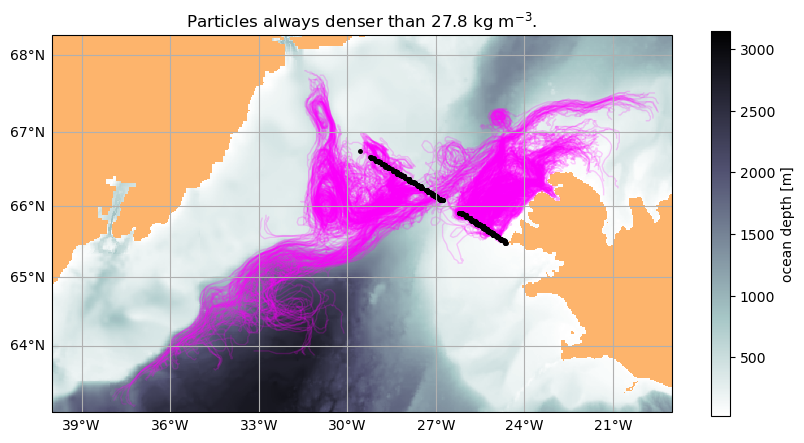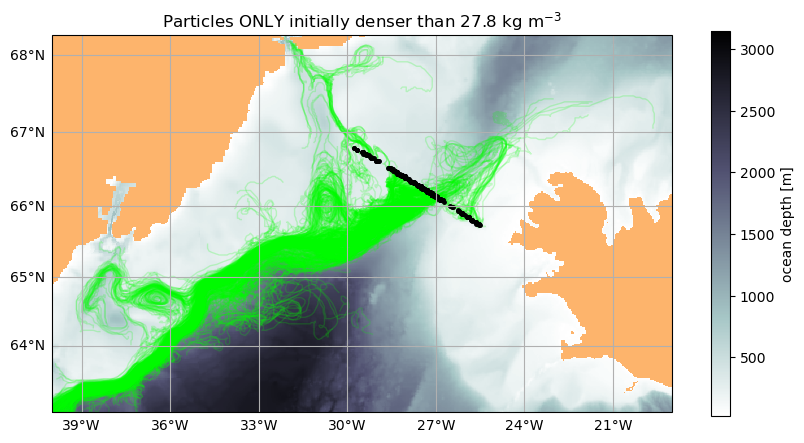## TS-diagrams¶

We can use TS-diagrams to investigate the hydrographic properties of the od_dense and od_mixed sets. For example, here we plot each oceandataset color-coded by particle depths separately (subplots a and b), then we plot them together on the same isopycnal contours (subplot c).

:

fig = plt.figure(figsize=(10, 10))

for i, (od_i, col, tit) in enumerate(zip(ods, colors, titles)):
plt.subplot(int('22'+str(i+1)))
ax = od_i.plot.TS_diagram(colorName='Z', alpha=0.5,
cmap_kwargs={'vmin': -2000, 'vmax': 0})
ax.set_title(chr(ord('a') + i)+') '+tit)

plt.subplot(212)
Tlim = [min([od_i.dataset['Temp'].min().values for od_i in ods]),
max([od_i.dataset['Temp'].max().values for od_i in ods])]
Slim = [min([od_i.dataset['S'].min().values for od_i in ods]),
max([od_i.dataset['S'].max().values for od_i in ods])]
for i, (od_i, col, tit) in enumerate(zip(ods, colors, titles)):
contour_kwargs={}
if i==0:
contour_kwargs['levels'] =[]
ax = od_i.plot.TS_diagram(color=col,
alpha=0.1,
Tlim=Tlim,
Slim=Slim,
contour_kwargs=contour_kwargs)
tit = '\n'.join(['c) ']+[f'{col}: {tit}' for _, (col, tit) in enumerate(zip(colors, titles))])
ax.set_title(tit)

plt.tight_layout()

Isopycnals: Computing potential density anomaly using the following parameters: {'eq_state': 'jmd95'}.
Isopycnals: Computing potential density anomaly using the following parameters: {'eq_state': 'jmd95'}.
Isopycnals: Computing potential density anomaly using the following parameters: {'eq_state': 'jmd95'}.
Isopycnals: Computing potential density anomaly using the following parameters: {'eq_state': 'jmd95'}.

/home/idies/miniconda3/envs/Oceanography/lib/python3.7/site-packages/oceanspy/plot.py:276: UserWarning: No contour levels were found within the data range.
CS = ax.contour(s.values, t.values, dens.values, **contour_kwargs)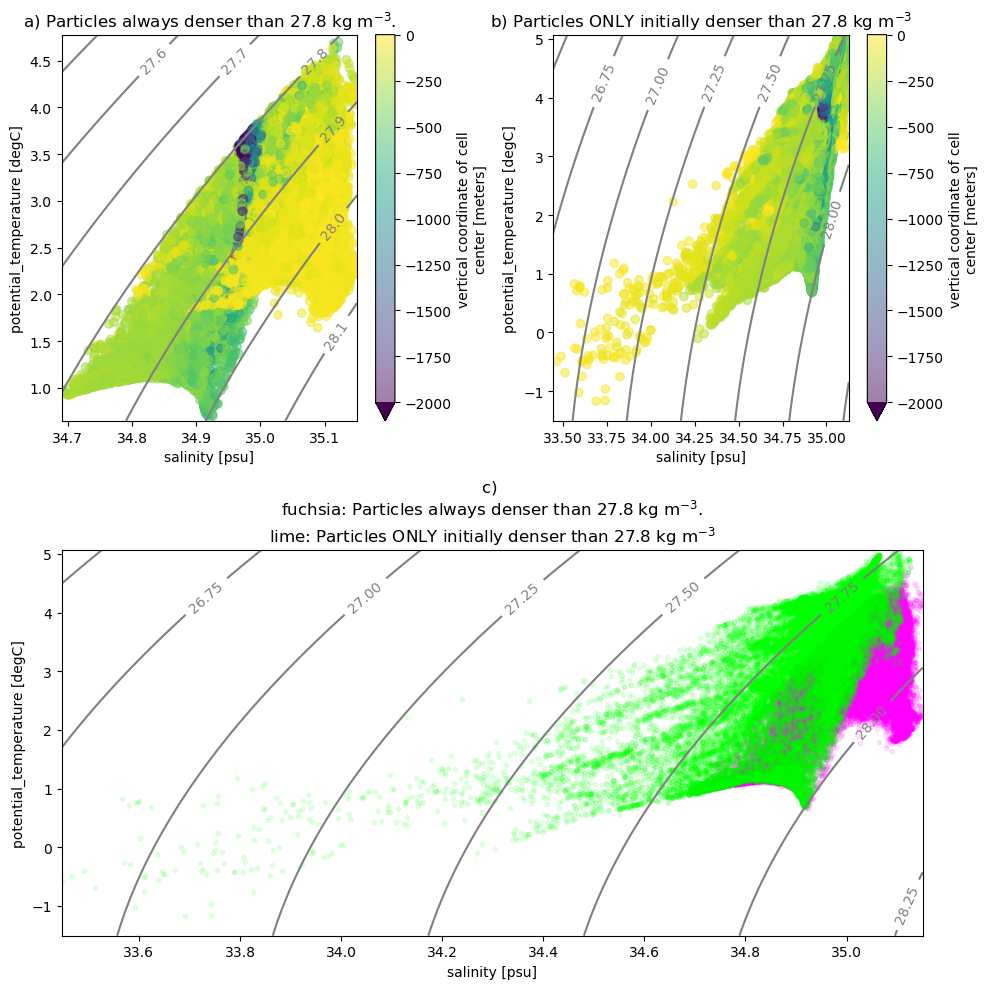## Time series¶

Now, we select 5 particles that were seeded in the overflow and became less dense along the path using the od_mixed set:

:

nparts = 5
step = np.ceil(len(od_mixed.dataset['particle'])/5)
od_5parts = ospy.OceanDataset(od_mixed.dataset.isel(particle=slice(None, None, int(step))))


Here is a plot of their trajectories:

:

ods = [od_dense, od_mixed]
colors = ['fuchsia', 'lime']
titles = [r'Particles always denser than 27.8 kg m$^{-3}$.',
r'Particles ONLY initially denser than 27.8 kg m$^{-3}$']

# Plot Depth
fig = plt.figure(figsize=(10, 5))
ax = od_eul.plot.horizontal_section(varName='masked_Depth', cmap='bone_r')
land_col = (253/255, 180/255, 108/255)
ax.background_patch.set_facecolor(land_col)
ax.set_extent([-40, -19, 63, 68])

# Plot trajectories
traj = ax.plot(od_5parts.dataset['XC'],
od_5parts.dataset['YC'],
linewidth=3,
transform=PlateCarree())

# Plot initial positions
i_pos = ax.plot(od_5parts.dataset['XC'].isel(time=0),
od_5parts.dataset['YC'].isel(time=0),
'k.', markersize=10,
transform=PlateCarree())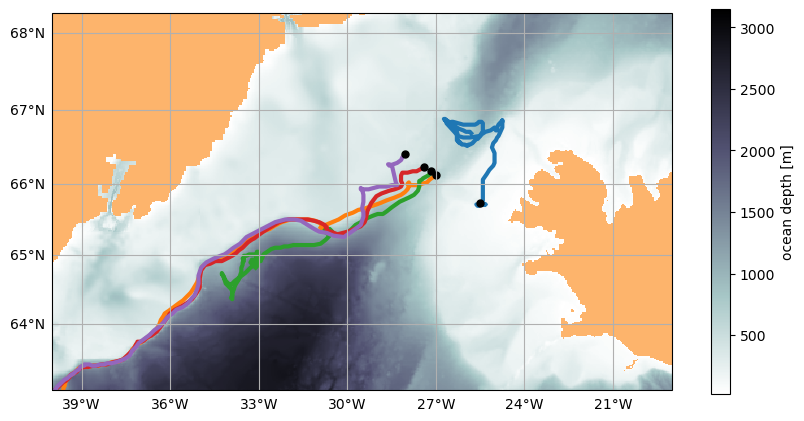Now we plot the change in potential density anomaly as a function of time of these five particles (1st plot below), and the potential density anomaly gradient (2nd plot). The rapid decrease in particle density corresponds to intense mixing and entrainment of lighter ambient water as the overflow descends into the Irminger Sea. Notice one particle on the Iceland shelf (in blue) is not part of the overflow and moves north then mixes.

:

varName = 'Sigma0'
fig, axes = plt.subplots(2, 1, figsize=(10, 10), sharex=True)
for i, ax in enumerate(axes.flatten()):
if i==1:
varName = f'd{varName}_dtime'
ax = od_5parts.plot.time_series(varName=varName, ax=ax, linewidth=2)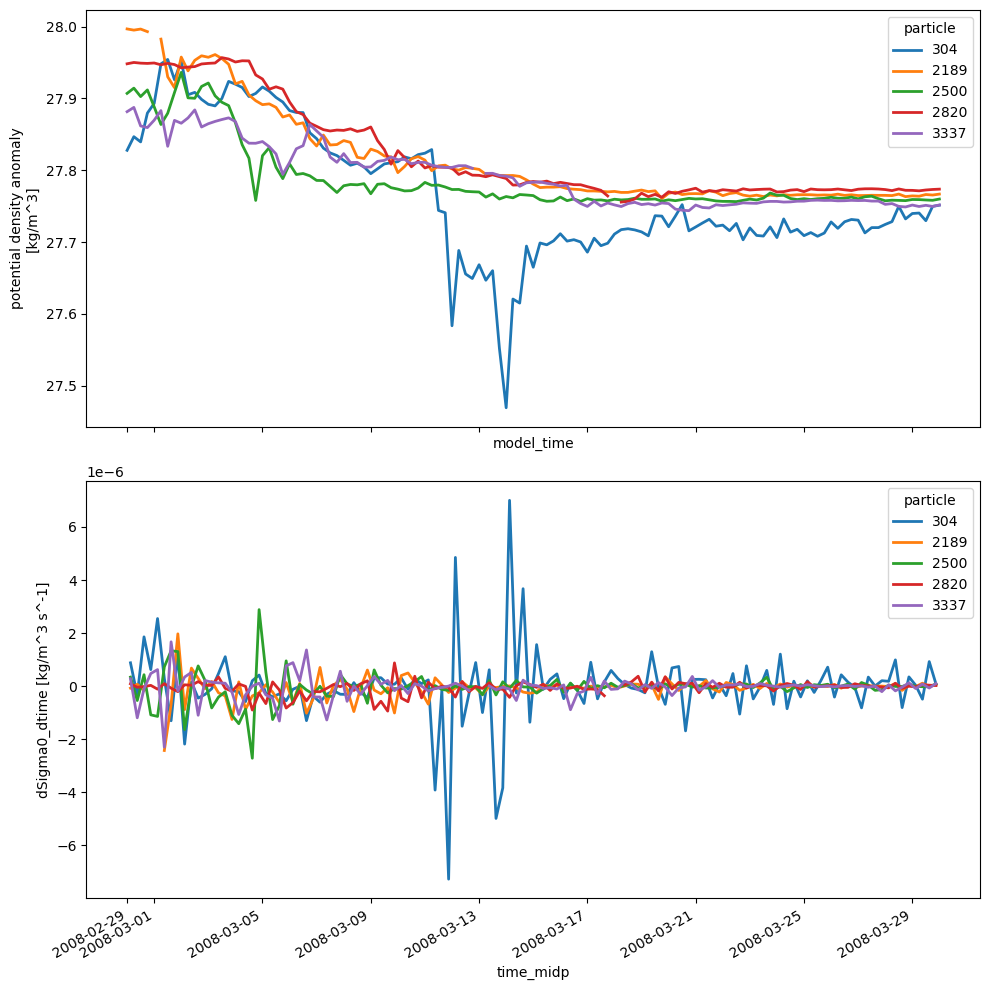The plots above can be easily customized for any of the extracted variables. For example, change varName = 'Sigma0' in the cell above with any of the variables printed below.

:

# Create table
table = {var: od_5parts.dataset[var].attrs.pop('long_name',
od_5parts.dataset[var].attrs.pop('description', None))
for var in od_5parts.dataset.variables
if 'time' not in var and 'particle' not in var}
print("\n{:>15}: {}\n".format('varName', 'label'))
for name, desc in sorted(table.items()):
print("{:>15}: {}".format(name, desc))


varName: label

Depth: ocean depth
S: salinity
Sigma0: potential density anomaly
Temp: potential_temperature
XC: longitude
XG: longitude
YC: latitude
YG: latitude
Z: vertical coordinate of cell center
Zl: vertical coordinate of upper cell interface
Zp1: vertical coordinate of cell interface
Zu: vertical coordinate of lower cell interface
momVort3: 3rd component (vertical) of Vorticity